#### 期刊菜单

Analysis of Vibration Characteristics Induced by Water Hammer of Ship’s Longitudinal Inclination and Balance System
DOI: 10.12677/APF.2020.104005, PDF, HTML, XML, 下载: 269  浏览: 587

Abstract: In the process of underwater navigation, it is necessary to move water in the longitudinal inclina-tion and balance tank frequently. Water hammer often occurs, which induces high-intensity tran-sient vibration and transient noise of the system. The transient load force is output through Flow Master, and the model of water transfer system is established in CAESAR II for time history analysis and calculation. At the same time, the influence of support height, support stiffness, fixing mode and support spacing on the vibration of longitudinal inclination and balance system is calculated and analyzed, which provides support for the subsequent three-dimensional design of pipeline system.

1. 引言

2. 水锤基本理论与数学模型

2.1. 水锤基本方程

2.1.1. 运动方程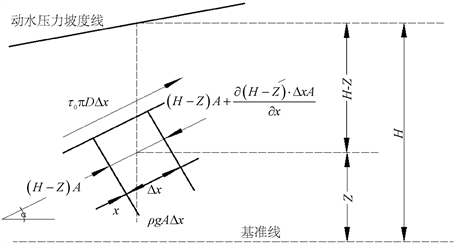Figure 1. Forces on small water bodies

$-\frac{\partial \left(H-Z\right)\cdot \Delta xA}{\partial x}-\rho g\Delta x\mathrm{sin}\alpha -{\tau }_{0}\pi D\Delta x=\rho A\Delta x\frac{\text{d}V}{\text{d}t}$ (2.1)

$\frac{\text{d}V}{\text{d}t}=\frac{\partial V}{\partial t}+\frac{\partial V}{\partial x}\frac{\partial x}{\partial t}=\frac{\partial V}{\partial t}+V\frac{\partial V}{\partial x}$ (2.2)

$g\frac{\partial H}{\partial x}+V\frac{\partial V}{\partial x}+\frac{\partial V}{\partial t}+\frac{4{\tau }_{0}}{\rho D}=0$ (2.3)

${\tau }_{0}=\frac{1}{8}\rho f{V}^{2}$ (2.4)

$\frac{\partial H}{\partial x}+\frac{V}{g}\frac{\partial V}{\partial x}+\frac{1}{g}\frac{\partial V}{\partial t}+\frac{fV}{2gD}|V|=0$ (2.5)

2.1.2. 连续性方程

$-\frac{\partial }{\partial x}\left(\rho AV\text{d}t\right)\text{d}x=\frac{\partial }{\partial t}\left(\rho A\text{d}x\right)\text{d}t$ (2.6)

$\frac{\partial \left(\rho A\right)}{\partial t}+\frac{\partial \left(\rho AV\right)}{\partial x}=0$ (2.7)

$-\frac{\partial V}{\partial x}=\frac{1}{A}\frac{\text{d}A}{\text{d}t}+\frac{1}{\rho }\frac{\text{d}\rho }{\text{d}t}$ (2.8)

$\frac{\partial H}{\partial t}+V\frac{\partial H}{\partial x}+\frac{{a}^{2}}{g}\frac{\partial V}{\partial x}=0$ (2.9)

V——管道中流体的流速(m/s)；

$\alpha$ ——水锤波波速(m/s)。

$\frac{\partial H}{\partial x}\ll \frac{\partial H}{\partial t}$ (2.10)

$\frac{\partial H}{\partial t}+\frac{{a}^{2}}{g}\frac{\partial V}{\partial x}=0$ (2.11)

3. 系统建模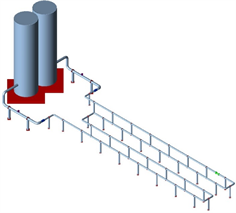Figure 2. Overall system modelFigure 3. Flange—valve model

4. 纵倾移水系统水锤激振时间历程分析Table 1. Displacement under maximum impact pressure

5. 管系振动影响因素研究

5.1. 支撑高度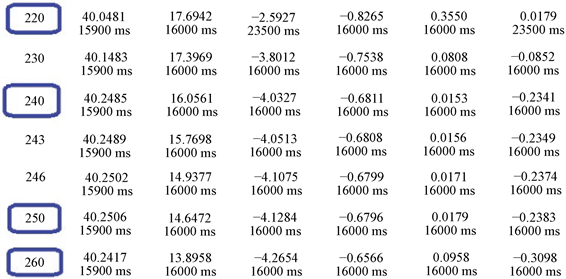Figure 4. Original calculation results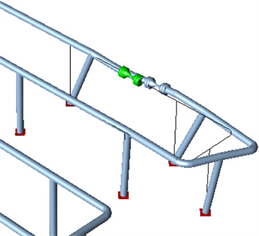Figure 5. Peak vibration displacement deformation of water hammer in front of valveFigure 6. Peak vibration displacement deformation of water hammer behind valve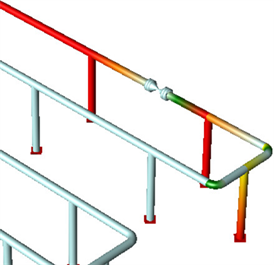Figure 7. Cloud chart of peak stress of water hammer in front of valve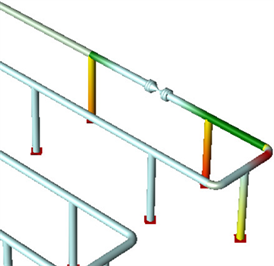Figure 8. Cloud chart of peak stress of water hammer behind valveTable 2. Maximum displacement of flange and support near valve at 520 mm heightTable 3. Maximum displacement of flange and support near valve at 1020 mm heightTable 4. Maximum displacement of flange and support near valve at 1520 mm height

5.2. 支撑刚度Table 5. Maximum displacement of flange and support near valve under 1000 N/cm stiffnessTable 6. Maximum displacement of flange and support near valve under 2500 N/cm stiffnessTable 7. Maximum displacement of flange and support near valve under 5000 N/cm stiffnessTable 8. Maximum displacement of flange and support near valve under 10000 N/cm stiffness

5.3. 固定方式Table 9. Maximum displacement of flange and support near the valve when fixed support is adoptedTable 10. Maximum displacement of flange and support near valve when original support is adopted

5.4. 支撑间隔Table 11. Maximum displacement of flange and support near valve with support interval of 4 mTable 12. Maximum displacement of flange and support near valve when original support is adopted

6. 小结

  周小红. 水锤现象及防护措施[J]. 冶金动力, 2016(7): 46-51.  郎梼, 鞠小明, 杨济铖. 不同类型阀门水锤特性计算比较[J]. 东北水利水电, 2015(7): 49-51+72.  杨晓红, 时兴波, 祝洪青, 等. 止回阀对给水系统水锤的影响[J]. 化工设备与管道, 2014, 51(6): 58-61.  冀健红, 高传昌, 刘新阳. 长距离多泵联合运行输水系统停泵水锤防护措施[J]. 南水北调与水利科技, 2014, 12(6): 112-115.  王鹤, 陆金龙. 压力供水管道试通水爆管原因分析及探讨[J]. 供水技术, 2014, 8(1): 42-47.